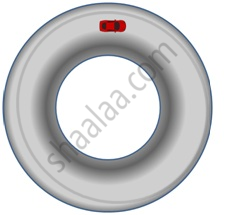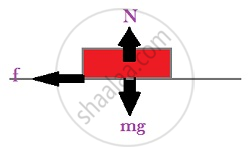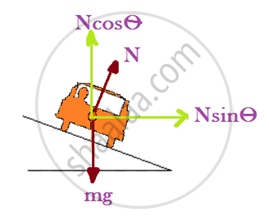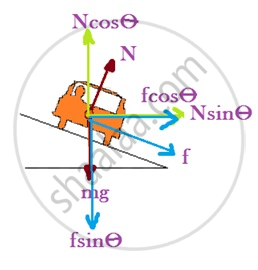# Examples of Circular Motion (Vehicle on a Level Circular Road, Vehicle on a Banked Road)

## Motion of a car on a level road

Three forces act on the car:
(i) The weight of the car, mg
(ii) Normal reaction, N
(iii) Frictional force, f

As there is no acceleration in the vertical direction
N – mg = 0
N = mgThe centripetal force required for circular motion is along the surface of the road, and is provided by the component of the contact force between road and the car tyres along the surface. This by definition is the frictional force
f =< μs N

According to the second law, the force f providing this acceleration is:
f_c=(mv^2)/R

hence, (mv^2)/r =< μs N
v^2 =< (μs N R)/m
= μs m g R/m
= μs R g
v =< sqrt (μs R g)
This is the maximum speed of a car in circular motion on a level road

## Motion of a car on a banked roadSince there is no acceleration along the vertical direction, the net force along this direction must be zero. Hence,
In the vertical direction (Y axis)
N cos ϴ = f sin ϴ + mg         ----------------- (i)

The centripetal force is provided by the horizontal components of N and f.
In horizontal direction (X axis)
f cos ϴ + N sin ϴ = (mv^2)/r             ------------- (ii)

Since we know that f = μs N
For maximum velocity, f = μs N
(i) becomes:
N cos ϴ = μs N sin ϴ + mg
Or, N cos ϴ – μs N sin ϴ = mg
Or, N = (mg)/(cos ϴ- μs sin ϴ)

Put the above value of N in (ii)
μ s N cos ϴ + N sin ϴ = (mv^2)/r
(μs m g cos ϴ)/(cos ϴ- μs sin ϴ) + (mg sin ϴ)/(cos ϴ- μs sin ϴ) = (mv^2)/r
(mg (sin ϴ + μs cos ϴ))/ (cos ϴ – μs sin ϴ) = (mv^2)/r

Divide the Numerator & Denominator by cos ϴ, we get
v^2= (R g (tan ϴ +μs))/(1- μs tan ϴ)
v = sqrt(( R g (tan ϴ + μs))/(1- μs tan ϴ))
This is the maximum speed of a car on a banked road.

Special case:
When the velocity of the car = v_0,

• No f is needed to provide the centripetal force. (μ_s =0)

• Little wear & tear of tyres take place.

v_o = sqrt(R g (tan ϴ))

Problem: A circular racetrack of radius 300 m is banked at an angle of 15°. If the coefficient of friction between the wheels of a race-car and the road is 0.2, what is the
(a) optimum speed of the race car to avoid wear and tear on its tyres, and
(b) maximum permissible speed to avoid slipping?
Solution.
R = 300m
ϴ = 15^o
μs = 0.2

v_o = sqrt (Rg tan ϴ) = sqrt(300 * 9.8 * tan 15^o) = 28.1 m/s

R = 30

vmax = sqrt ((R g (tan ϴ + μs)) /(1- μs tan ϴ))

= sqrt(( 300 * 9.8 * (0.2 + tan 15o))/(1-0.2tan15o))

= 38.1 m/s

If you would like to contribute notes or other learning material, please submit them using the button below.